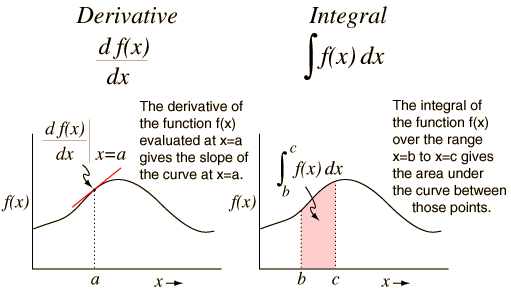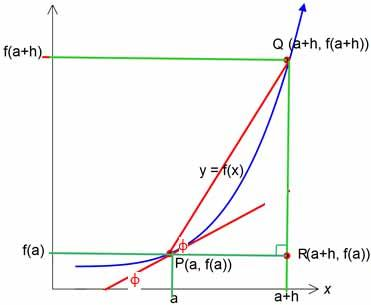COURSE
EXAM
STUDY MATERIALS
BOOKS
PDFs

Click Question to Get Free Answers >

# RD Sharma Class 11 and Chapter Derivatives Maths Solutions

## Maths Class 11 and Chapter Derivatives RD Sharma SolutionsFilter
Filters :
CLASSES
•
•
•
•
•
•
•
CHAPTERS
•
• 23 More

# RD Sharma Class 11 Solutions - Chapter 30 Derivatives

In mathematics, Derivative simply represents the rate of change of any function with respect to another variable. Derivatives are important part of calculus and differential equations to find the solution of a problem. When a mathematician observes a shifting or dynamical system in order to find out the rate of change of any variable in study, derivatives are incorporated into the differential equation, by the utilization of the integration techniques to obtain a function. It is an essential concept to help predict the behavior of the any system under diverse environmental settings.In Geometry, the derivative of a function can be taken as the slope of the tangent line at a point on a graph. The calculations of the derivatives are derived from the slope formula of a straight line, apart from that a limiting operation must be used for curves. In writing, the derivatives are expressed "dy over dx". It represents difference in y divided by the difference in x. Derivative can be expressed as: dydx  here d is not a variable, and for that reason cannot be annulled out.

## Real-world applications of derivatives

During the days of Sir Isaac Newton, one of the major problems around the world was the navigation in the sea. Shipwrecks were quite frequent due to the captain’s lack of understanding of the coordinate system. There were also lack of knowledge of movement of planets and stars with respect to each other. Therefore, during those days, mathematicians worked had for the development of basic calculus in order to improve the navigation so as the mariners needed not to relay on the stars. The advancement in calculus helped to improve such understanding and solve a plethora of real-world problems.

Delineated below are some interesting applications of Derivatives:

1. To determine the maximum and minimum values of particular functions (e.g. cost, strength, amount of material used in a building, profit, loss, etc.).

2. One of the important applications of derivatives is in Newton's method. It helps in finding the zeros (roots) of a function.

3. Derivatives are met in many engineering and science problems, especially when modeling the behavior of moving objects.

## Derivatives: Class 11 Maths RD Sharma Solutions

RD Sharma Class 11 Solutions - Chapter 30, Derivatives, will be exclusively focusing on the computation of derivatives. Derivative is an important topic of calculus. With rigorous practice, one can master the mathematical concepts pertaining to Chapter 30, Derivatives. You will also be learning about various applications of derivatives, that’ll be helpful for you to relate the chapter to its real-world applications. Given RD Sharma Class 11 Solutions - Chapter 30 Derivatives covers various important topics as delineated below:

1. Motion in Straight Line

2. Geometrical Meaning of Derivative at Point

3. Monotonicity

4. Concept of Local Maxima and Local Minima

5. Global Maxima and Minima

6. Rolle Theorem and Lagrange Mean Value Theorem

7. Solved Examples of Applications of Derivatives

## Topics and Subtopics of RD Sharma Class 11 Solutions Chapter 30 Derivatives

Get here fully-solved RD Sharma Class 11 Solutions Chapter 30 Derivatives in video tutorial format. Each exercise is introduced with theory including illustrative examples. Find here fully solved exercises and various practice questions. It will be beneficial for you to test your understanding of all the topics covered in this chapter. Given RD Sharma Class 11 Solutions Chapter 30 Derivatives is in self-explanatory video tutorial format offering you the ease of learning on your mobile or personal computer.  The video solutions have been created in easy to understand language. Besides following the regular methods of solving a problem, the expert mathematicians from Doubtnut have also added special suggestions to make you fall in love with the subject. All the exercises have been covered with step-by-step detailed explanation. The solutions are prepared keeping in mind the latest CBSE board curriculum. Make sure to go through the RD Sharma Class 11 Solutions Chapter 30 Derivatives thoroughly.

### Geometric Interpretation of Derivatives

Let y = f(x) be a function, and let P (a, f (a)) and Q (a+h, f (a+h)) be two points on the graph of the function that are close to each other. This graph is shown below:Joining the points P and Q with a straight line gives us the secant on the graph of the function. And in the ∆PQR the gradient of the line is given by

m = f (a=h) – f (a) a=h-a = f a+h-f ah ,

In limiting process, i.e. as Q approaches P, h becomes really small, almost close to zero.

So, m =change in ychange in x

= yx  = f a+h-f (a)h

yx   = f a+h-f(a)h

As Q → P the chord/secant PQ tends to be a tangent at P for the curve y = f(x). Thus, the limiting becomes the slope of the tangent at P for y = f(x).

We denote yx       = dydx  ,

And, h→0  fa+h-f(a)h   = f’ (a)

Therefore dydx  = f’ (a) = m

m as we’ve seen is the slope or gradient of the tangent at P for f(x).

f'(a) = tan Ɵ = slope of f(x) at x = a.

## Here are some RD Sharma solved solutions for you

RD Sharma Illustration 1: The function y = 2x2 – log |x| is monotonically increasing for values of x ≠ 0 satisfying the inequalities ……. and monotonically decreasing for values of x satisfying…..

Solution: The given function is y = 2x2 – log |x|.

x ∈ (-1/2, 0) ∪ (1/2, ∞), x ∈ (-∞ , -1/2) ∪ (0, ½)

Here, y = 2x2 – log x         , x >0

2x2 – log (-x)   , x < 0

So this means that:

dy/dx = 4x – 1/x, x >0

= 4x – 1/x, x < 0

Hence, dy/dx = 4x2 – 1/x, x ∈ R – {0}

= (2x -1) (2x +1)/x

Therefore, it is increasing when x ∈(-1/2, 0) ∪ (1/2, ∞)

And decreasing when x ∈ (-∞, -1/2) ∪ (0, ½).

RD Sharma Illustration 2: The maximum value of

(cos α1). (cos α2)…. (cos αn), under the restrictions 0 ≤ α1, α2,… , αn ≤ π/2

(cot α1). (cot α2)…. (cot αn) = 1 is

1. 1/2n/2                                                            2. 1/2n

3. 1/2n                                                            4. 1

Solution: Given cot α1. cot α2….....cot αn = 1

Then, cos α1/sin α1 . cos α2/sin α2 . cos α3/sin α3 ….. cos αn/sin αn = 1.

Hence, cos α1. cos α2…. cos αn = k …….. (1)

and sin α1. sin α2……. sin αn = k …….. (2)

by multiplying equations (1) and (2) we get,

(cos α1. cos α2…… cos αn) x (sin α1. sin α2……. sin αn) = k2

Then k2 = 1/ 2.2.2…….. n times . (2 sin α1. cos α1) (2 sin α2. cos α2) (2 sin α3. cos α3)…………  (2 sin αn. cos αn)

Hence, k2 = 1/2n (sin 2α1).(sin 2α2)…….. (sin 2αn)

≤ 1/2n sin 2αi ≤ 1 for all 1≤ i ≤ n

Hence, k ≤ 1/2n/2. Hence the correct option is (1).

RD Sharma Illustration 3: If f(x) = xa log x and f(0) = 0, then the value of a for which the Rolle’s theorem can be applied in [0,1] is

1. -2                                                        2. -1

3. 0                                                         4. ½

Solution: In order to satisfy the Rolle’s Theorem, the function needs to be continuous in [0, 1].

So, lim x→0+ f(x) = f(0)

lim x→0+ log x/ x-a = 0.

lim x→0+ (1/x)/-ax-a-1 = 0.

lim x→0+ xa/ a = 0.

This clearly implies that a > 0.

Now, ∀ a > 0, f(x) is differential in (0, 1) and f (1) = 0 = f (0).

## Get Started with Doubtnut Now!

At Doubtnut, we strive to facilitate the easy and rightful access to quality study materials to students of all age groups. You can also download our App “Doubtnut” from Google play store. With the highest 4.4 play store rating, Doubtnut App is the student’s favorite e-learning destination to prepare for competitive exam like IIT-JEE and board examination. Keep visiting doubtnut.com for more interactive study material